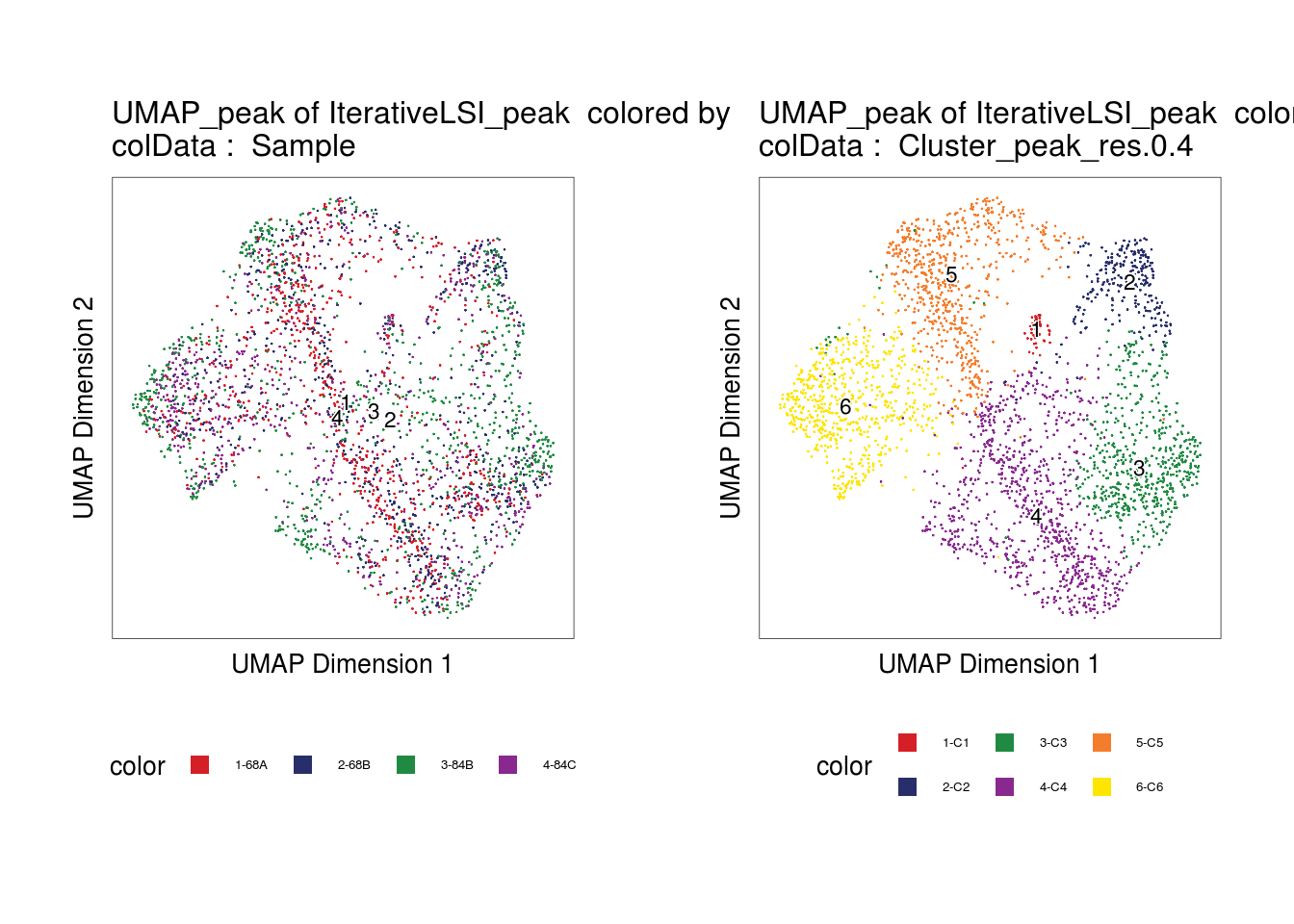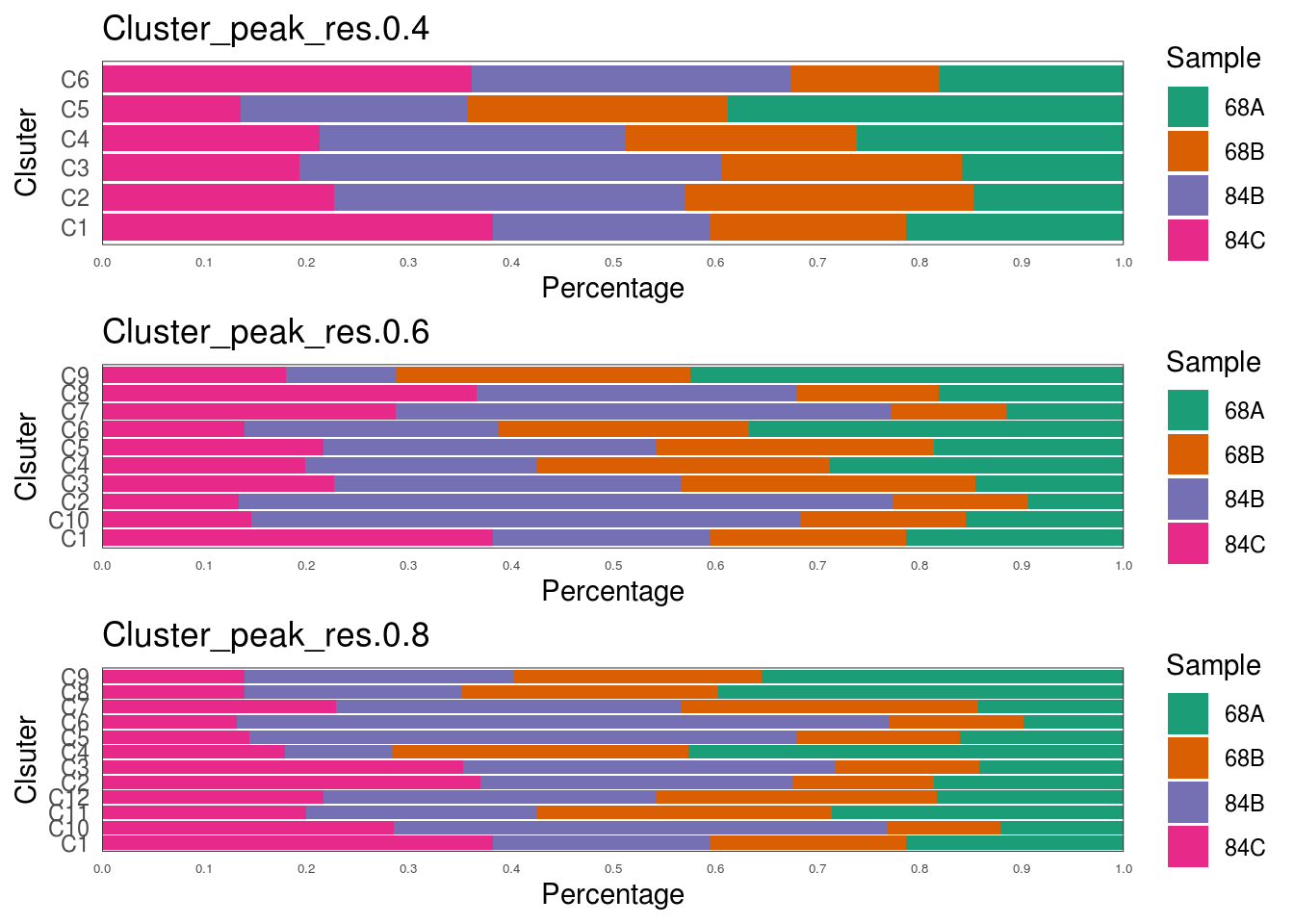# Chapter 14 Check Batch Effect Introduced by Individuals

``````library(ArchR)
library(tidyverse)
library(magrittr)
set.seed(1)``````

## 14.1 Description

• Check whether exist significant batch effect introduced by individuals
• Correct batch effect by Harmony
• Explore for both rounds of clustering. If exits batch effect, correct both rounds

## 14.2 Set env and load arrow project

``````## Section: set default para
##################################################

##################################################

## 14.3 UMAP to check the batch effect for the second round clustering

``````name.ls <- c("Sample", "Cluster_peak_res.0.4")

p.ls <- name.ls %>% map(~ {
plotEmbedding(
ArchRProj = proj,
colorBy = "cellColData",
name = .x,
embedding = "UMAP_peak"
)
})``````
``````## ArchR logging to : ArchRLogs/ArchR-plotEmbedding-265f1f863b75-Date-2021-07-26_Time-21-01-17.log
## If there is an issue, please report to github with logFile!``````
``## Getting UMAP Embedding``
``## ColorBy = cellColData``
``## Plotting Embedding``
``````## 1
## ArchR logging successful to : ArchRLogs/ArchR-plotEmbedding-265f1f863b75-Date-2021-07-26_Time-21-01-17.log
## ArchR logging to : ArchRLogs/ArchR-plotEmbedding-265f2ebf4e0a-Date-2021-07-26_Time-21-01-20.log
## If there is an issue, please report to github with logFile!
## Getting UMAP Embedding
## ColorBy = cellColData
## Plotting Embedding
## 1
## ArchR logging successful to : ArchRLogs/ArchR-plotEmbedding-265f2ebf4e0a-Date-2021-07-26_Time-21-01-20.log``````
``ggAlignPlots(p.ls[], p.ls[], type = "h")``## 14.4 Calculate the individual percentages per cluster

``````df <- as.data.table(proj@cellColData)
sel.meta <- c("Sample", str_c('Cluster_peak_res.', c(0.4, 0.6, 0.8)))

df <- df[, sel.meta, with = FALSE]

p.ls <- df[, 2:4] %>% imap(~ {
freq1 <- df[, .N, keyby = .(.x, Sample)]
freq1[, total := sum(N), by = .(.x)]
freq1[, ratio := N / total]

linesize = .35
fontsize = 8

ggplot(freq1, aes(fill = Sample, y = ratio, x = .x)) +
geom_bar(position = "stack", stat = "identity") +
scale_fill_brewer(palette = "Dark2") +
xlab('Clsuter') +
ggtitle(.y) +
scale_y_continuous(breaks = seq(0, 1, 0.1),
expand = c(0, 0),
name = 'Percentage') +
theme_bw() +
theme(
panel.grid.major.x = element_blank(),
panel.grid.major.y = element_blank(),
panel.grid.minor = element_blank(),
strip.background = element_blank(),
panel.border = element_rect(size = linesize),
axis.ticks = element_blank(),
axis.text.x = element_text(size = 5)
) +
coord_flip()
})

ggAlignPlots(p.ls[], p.ls[], p.ls[], type = "v")``````## 14.5 Correct batch effect using harmony

If exits significant batch effect

``````# if correct the tile matrix
ArchRProj = proj,
reducedDims = "IterativeLSI_tile",
name = "Harmony_tile",
groupBy = "Sample"
)

# if correct the peak matrix